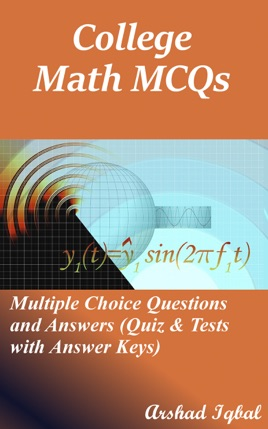• 4,49 €

## Publisher Description

College Math Multiple Choice Questions and Answers pdf: MCQs, Quizzes & Practice Tests. College math quiz questions and answers pdf with practice tests for online exam prep and job interview prep. College math study guide with questions and answers about application of basic identities, double angle identities, functions and limits, fundamentals of trigonometry, matrices and determinants, number system, partial fractions, permutations, combinations and probability, quadratic equations, sequences and series, sets, functions and groups, trigonometric functions and graphs, trigonometric identities, trigonometric ratios of allied angles.
College math MCQ questions and answers to get prepare for career placement tests and job interview prep with answers key. Practice exam questions and answers about math, composed from college math textbooks on chapters:

Application of Basic Identities Practice Test: 20 MCQs
Double Angle Identities Practice Test: 19 MCQs
Functions and Limits Practice Test: 125 MCQs
Fundamentals of Trigonometry Practice Test: 78 MCQs
Matrices and Determinants Practice Test: 26 MCQs
Number System Practice Test: 68 MCQs
Partial Fractions Practice Test: 36 MCQs
Permutations, Combinations and Probability Practice Test: 69 MCQs
Quadratic Equations Practice Test: 76 MCQs
Sequences and Series Practice Test: 79 MCQs
Sets, Functions and Groups Practice Test: 30 MCQs
Trigonometric Functions and Graphs Practice Test: 42 MCQs
Trigonometric Identities Practice Test: 125 MCQs
Trigonometric Ratios of Allied Angles Practice Test: 10 MCQs

Mathematics interview questions and answers on addition of matrix, adjoint and inverse of square matrix, algebra and trigonometry, arithmetic mean, arithmetic mean (am), arithmetic progression, basic function, basic trigonometric identities, basic trigonometry formulas, bi-conditional, binary operation, circular permutation, college algebra: trigonometric function, college math, column matrix, combinations, complementary combination.
College math test questions and answers on complex numbers, composition of functions, concept of limit of function, cube roots of unity, domains and ranges, double angle identities, even functions, exponential equations, exponential function, finding inverse function, formation of equation whose roots are given, fourth root of unity, fundamental identities, geometric mean, geometric progression (GP), groups in maths, harmonic mean, harmonic progression (HP).
College math exam questions and answers on homogeneous linear equations, hyperbolic functions, implication or conditional, infinite geometric series, introduction of partial fractions, introduction of sequences and series, permutations, combinations and probability, introduction to functions and limits, introduction to matrices and determinants, functions and groups.
College math objective questions and answers on inverse functions, inverse of a function, linear and quadratic function, linear function, nature of roots of quadratic equation, notation and value of function, number systems: sets, odd functions, online math learning, operation on sets, operation on three sets, parametric functions, period of trigonometric functions, polynomial function, properties of real numbers, radian to degree conversion, radians to degrees, rational fractions, row matrix, sequences and series, series, sigma notation, sine cosine tangent, skew-symmetric matrix.
Math certification prep questions on solution of a quadratic equations, sum of n terms of a geometric series, symmetric matrix, synthetic division, tangent and cotangent functions, the relation, trig identities, trigonometric formulas, trigonometric function, trigonometric identities, trigonometric ratios of allied angles, trigonometry basics, trigonometry problems, triple angle identities, when q(x) has non-repeated irreducible quadratic factors, when q(x) has non-repeated linear factors, when q(x) has repeated linear factors for competitive exams preparation.